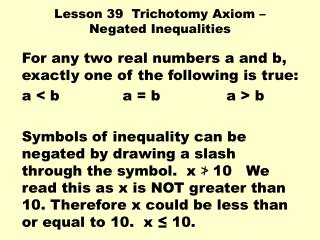DownloadDownload PresentationLesson 39 Trichotomy Axiom – Negated Inequalities

Lesson 39 Trichotomy Axiom – Negated Inequalities

Download PresentationLesson 39 Trichotomy Axiom – Negated Inequalities

- - - - - - - - - - - - - - - - - - - - - - - - - - - E N D - - - - - - - - - - - - - - - - - - - - - - - - - - -
Presentation Transcript

1. Lesson 39 Trichotomy Axiom – Negated Inequalities For any two real numbers a and b, exactly one of the following is true: a < b a = b a > b Symbols of inequality can be negated by drawing a slash through the symbol. x ≯ 10 We read this as x is NOT greater than 10. Therefore x could be less than or equal to 10. x ≤ 10.

2. Draw a number line & graph the solution: • x ≮ 2 Advanced Ratio Problems